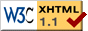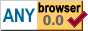Micropal

# Micropal

2021-04-30

## Introduction

Micropal is a design that combines 8-bit color values with a small lookup table to enable 12-bit RGB colors (4 bits each for red, green and blue). Traditionally, graphics hardware that uses color lookup tables has used the color value purely as an index into the lookup table, with the lookup table providing all the actual RGB bits. Micropal instead uses the 8-bit color value to provide the least significant RGB bits, with the lookup table providing only the 4 most significant bits. This reduces the required hardware to extend color capability beyond fixed 256-color palettes, at the cost of not being able to freely choose all the RGB bits.

## Motivation

One of my hobbies is designing computers that can be built from simple electronic parts. Among the choices to be made in such a design is how to do graphics. There are numerous options, from 1-bit monochrome graphics to true color graphics where the image data directly encodes the red, green, and blue value for a pixel. Somewhere in between those exists the possibility to use one byte, 8 bits, to encode the color information for a pixel. This turns out to be not quite enough to get satisfying results (see Comparison for some examples).

Historically, computers have enabled better colors for 8-bit graphics by using the pixel value as a key in a lookup table that holds the actual color information. VGA, for example, has a lookup table that provides 6 bits each for red, green, and blue, for a total of 262,144 possible colors (compared to 8 bits' 256 possibilities). However, when building a computer from invidual integrated circuits, 18 bits is not a very nice quantity to work with. 16 bits would be easier, and can produce very good results. However, that's still two 8-bit memory ICs (or a 16-bit one, which tend to be more expensive) plus a couple of 5-bit DACs (and maybe a 6-bit one). Can we get good results with less?

Micropal reduces the required hardware to:

• A single memory IC, which needs to provide 256 addresses and 4 bits per address.
• 3x 4-bit digital-to-analog converters to convert the digital color information to an analog signal.

This page showcases some of the results that can be obtained with Micropal. I think it occupies a useful position between 8 bits per pixel without a lookup table and 8 bits per pixel with a lookup table that requires multiple ICs.

## 12-bit Color

The key idea in Micropal is to use the 8-bit color value not just as an index into the color lookup table, but also to provide 8 of the least significant bits of the RGB value. This allows us to get 12 bits of color information with the addition of a 4-bit lookup table, instead of a 12-bit lookup table that would otherwise be needed. The downside of this approach is that 8 of the 12 bits are not freely assignable, but since they are the least significant bits, we can presumably tolerate some error there.

In practice, the optimal 256-color palette for an image often includes many colors with the same most significant bits and many combinations of the least significant bits. This is exactly the scenario in which Micropal can produce good results. Micropal's weakness is images where all combinations of most significant RGB bits are roughly equally present and compete for the same combinations of less significant bits. In such cases, Micropal's results are similar to those of fixed 256-color palettes.

## Examples

This is an image from Wikimedia Commons for Terragen, a program which generates natural-looking scenery:Converted to 256 colors with a palette that can be represented by Micropal, the image might look like this:Or like this, without dithering:Here is the same image, scaled down to 320x180 pixels and the Micropal palette:The same 320x180 pixels image, but doubled in width and height to be more similar in size to the original:## Comparison

Here are some side-by-side comparisons of Micropal with various other 8-bit color schemes. All images are 320 pixel wide versions of the test image, 256 colors, with no dithering. In each case, Micropal is on the right.

The image on the left is an optimal 256-color palette for the image, as generated by GIMP 2.10.8. This uses 8 bits each for red, blue, and green, freely selectable for each palette entry (as compared to Micropal's 4 bits for each component, with restrictions on how they can be assigned).

 Optimal MicropalRGB332: This uses 3 bits for red and green and 2 bits for blue, giving a total of 8 red levels, 8 green levels, and 4 blue levels.

 RGB332 MicropalA slight modification of RGB332. This uses 3 bits each for red, green, and blue. The least significant bit for blue is computed from the other bits: if red's most significant bit is 1 and red's least significant bit is 0, blue's least significant bit is 1. In all other cases, blue's least significant bit is 0.

 Modified RGB332 MicropalA palette that divides red, green, and blue into 6 equal steps each, for a total of 216 different colors.

 6x6x6 MicropalThe VGA default palette. This palette consists of the 16 colors of the CGA palette (2-bits each for red, green, blue, intensity), 16 grayscale values, and 224 entries that cycle through colors by hue, saturation, and lightness.

 VGA default palette MicropalThe Windows 95 256 colours palette from this Lospec entry.

 Windows 256 colors Micropal## Code

The examples shown here were generated by running a script to generate a palette for an image, then having GIMP convert the image to using that palette. The script reads its input in the form of an ASCII PNM (P3) file and generates a GIMP palette (.gpl) file. The choice of colors is not guaranteed to be optimal, but it's good enough to get an idea of what Micropal can do. The script is here:

``````
from __future__ import division
from __future__ import print_function

# Read PNM file, pick colors that can be represented by Micropal, print
# out GIMP palette with those colors.

import sys

class Image(object):
def __init__(self, w, h, data):
self.width = w
self.height = h
self.data = data

def getpixel(self, x, y):
base = (y * self.width + x) * 3
return (data[base], data[base + 1], data[base + 2])

if line != 'P3':
raise Exception('Need P3 file, got %s' % (line,))

while True:
if len(line) > 0 and line != '#':
return line

def get_pieces():
for line in f:
for piece in line.split():
if scale == 255:
yield int(piece)
else:
yield int(float(piece) / scale * 256)

size = width * height * 3
data =  * size
inator = get_pieces()
for i in range(size):
data[i] = inator.next()

return Image(width, height, data)

def simple_colormap():
# A simple colormap which does not consider the image data at all.
colormap = []
for b in range(4):
for g in range(8):
for r in range(8):
mr = r & 1
mg = g & 1
mb = b
colormap.append(((mr << 7) | (r << 4),
(mg << 7) | (g << 4),
(mb << 6) | (b << 4)))
return colormap

def colormap_from_image(img):
# Micropal provides red, green, blue (RGB) colors with 4 bits for each
# of the components. However, the bits are not freely assignable.
#
# The bits are divided into major bits and minor bits. The major
# bits are the most significant bit of red, the most significant
# bit of green, and the most significant 2 bits of blue. The minor
# bits are the 3 least significant bits of red and green and the 2
# least significant bits of blue.
#
# Color is then encoded as follows:
#
#   rrrgggbb rgbb
#   -------- ----
#    minor   major
#
# where rrrgggbb is the color number, and rgbb is stored in a lookup
# table indexed by color number.
#
# Every combination of minor bits always exists in the palette.
# For every combination of minor bits, exactly one combination of
# major bits exists in the palette.
#
# The task of this function, then, is to assign exactly one major
# color to every minor color. The better a job this function does,
# the closer to the original the image will look after conversion
# to the resulting colormap.
#
# Algorithm
#
# Getting an optimal assignment of minor colors to major colors is an
# Interesting Problem. The algorithm used here is not guaranteed to
# be optimal, but it runs in a reasonable amount of time and produces
# good results on a number of images I have tried it on.
#
# We assign a value to each of the minor colors based on how many pixels
# use that minor color.
#
# We assign a budget to each major color based on how many pixels use that
# major color.
#
# Then we auction off the minor colors, in an order that is deterministic
# but spread out through the color space. For each minor color, all
# major colors that want it place a bid, and the highest bidder wins.
#
# The function that determines the bid is currently relatively simple.
# One way it might be improved is by considering nearby colors and
# whether or not they are still available. For example, we may be willing
# to bid more on a minor color if we wanted a neighboring minor color,
# but lost the bid.
#
# It may also be beneficial to allow trading colors after the initial
# auction.

# First, we create some lookup tables.
#
# Bits are arranged from least significant to most significant.
# rgb is the 12-bit red, green, blue number: rrrrggggbbb.
# maj is the major color number. It consists of bits r...g...bb..
# c is the minor color number. It consists of bits .rrr.ggg..bb
#
# count[maj][c] is the number of occurrences of each color in the image.
# major[maj] is the budget for each major color. Initially, this is the
# number of occurrences of the major color in the image.

count = []
for i in range(16):
count.append( * 256)
major =  * 16
for color in colors_in_image(img):
rgb = nearest_rrrrggggbbbb(color)
maj = ((rgb & 8) >> 3) | ((rgb & 128) >> 6) | ((rgb & 3072) >> 8)
c = (rgb & 7) | ((rgb & 0x70) >> 1) | ((rgb & 0x300) >> 2)
major[maj] += 1
count[maj][c] += 1

def bid(m, c):
if major[m] < 1 or count[m][c] == 0:
return 0
total = 0
for i in range(256):
total += count[m][i]
return count[m][c] * major[m] / total

# We cycle through the colors so that the next color to be auctioned is never
# next to the previous color that was auctioned.
colormap = [(0, 0, 0)] * 256
c = 54
for i in range(256):
# 61 and 256 are relative primes, which guarantees that we will visit every
# minor color in 256 steps.
c = (c + 61) & 0xff
best = (0, 0)
for m in range(16):
b = bid(m, c)
if b > best:
best = (b, m)
colormap[c] = (((best & 1) << 7) | ((c & 7) << 4),
((best & 2) << 6) | ((c & 0x38) << 1),
((best & 0x0c) << 4) | ((c & 0xc0) >> 2))

return colormap

def colors_in_image(img):
for i in range(0, len(img.data), 3):
yield (img.data[i], img.data[i + 1], img.data[i + 2])

def nearest_rrrrggggbbbb(rgb):
return (to4bit(rgb) |
(to4bit(rgb) << 4) |
(to4bit(rgb) << 8))

def to4bit(c):
c = (c + 7) // 16
if c >= 16:
return 15
else:
return c

def main():